# HUBUNGAN ANTARA TINGKAT INTELEGENSI (IQ) DAN MOTIVASI BELAJAR DENGAN PRESTASI AKADEMIK SISWA DI SMA NEGERI 2 TANGERANG

SETIAWAN, GALIH (2014) HUBUNGAN ANTARA TINGKAT INTELEGENSI (IQ) DAN MOTIVASI BELAJAR DENGAN PRESTASI AKADEMIK SISWA DI SMA NEGERI 2 TANGERANG. S1 thesis, Universitas Negeri Jakarta.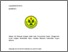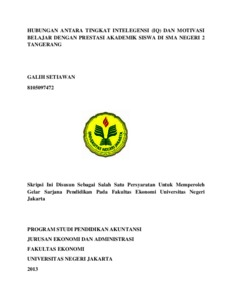Preview
Text
COver.pdf

Download (0B) | Preview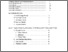Preview
Text
Table_Of_Content.pdf

Download (0B) | Preview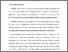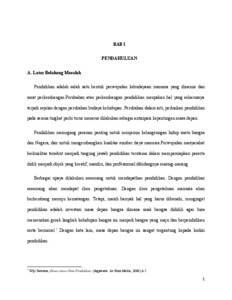Preview
Text
Chapter 1.pdf

Download (0B) | PreviewText
Chapter 2.pdf
Restricted to Registered users only

Download (0B)Preview
Text
Chapter 3.pdf

Download (0B) | PreviewText
Chapter 4.pdf
Restricted to Registered users only

Download (0B)Preview
Text
Chapter 5.pdf

Download (0B) | Preview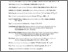Preview
Text
Bibiliography.pdf

Download (0B) | Preview

## Abstract

GALIH SETIAWAN. Relationship Between Intelligence Quotient ( IQ ) and Motivation Learning with Student Academic Achievement SMAN 2 Tangerang . Thesis , Jakarta: Accounting Education Program. Department of Economics and Administration , Faculty of Economics , State University of Jakarta.Januari 2014. Academic achievement is desire and expectations of all parties, both the school and the students themselves , however, achievement is not necessarily obtained by all students . Therefore , the school requires appropriate strategies to improve academic achievement , including through the IQ factor and motivation to learn . IQ and achievement requires considerable motivation to learn as a factor to support the academic achievement of students . This study aims to determine the relationship between the first , the relationship between intelligence quotient ( IQ ) and motivation to learn the academic achievement of students of SMAN 2 Tangerang . The data used are secondary and primary data . Data were analyzed with descriptive statistics and parametric statistics include correlation , coefficient of determination , t test , F test and regression . From the calculation , note the significant value of Y over 0,200 X sebesar . Thus the significance values > 0,005 sigifikansi level so that it can be concluded that the Y over the X 1 is normal . For a significance value Y over 0,200 X sebesar . Thus the significance values > 0,005 sigifikansi level so that it can be concluded that the Y over the X 1 is normal . Then , the results of statistical calculation shows the regression equation y = 2.558 + 0.006 X 2 adalah very significant , because the calculated F value ( 22.435 ) > = 0.05 , which is 3.17 .αF table at Meanwhile, to 1 test the linearity regression calculated F value of 29.514 , while = 0.05 with 26 df numerator andαthe value of the F - table at denominator df 1 is 249.453 . Therefore, the value of the F - count < F - table , the regression equation of academic achievement with intelligence quotient ( IQ ) is linear . The coefficient of determination ( ry1 ² ) = 0.286 , which means 28.6 % of the variation can be explained by the academic achievement variasiintelligence quotient ( IQ ) through a regression equation y = 2.558 + 0.006 X . To test the significance of the regression coefficient of Y over F - =αcalculated value X 2 1 diperoleh 22.375 while the value of F table at 0.05 is 3.17 . From the calculation it is known that the calculated F value ( 22.375 ) > F table value ( 3.17 ) , thus indicating that the regression equation Y Y = 2.719 + 0.007 X adalah very significant . As for the linearity test regression is known that the value of F obtained ( 20.004 ) < = 0.05 with 26 df numerator andαF table value at denominator df 1 ( 249.453 ) . With these results, the form of the regression equation y = 2.719 + 0.007 X is linear . The result significance of the correlation coefficient calculation obtained thit = 4.730 > table = 1.68 at α = 0.05 it can be concluded that the correlation coefficient between motivation learning withacademic achievement is very significant . The coefficient of determination ( ry2 ² ) = 0.285 which means that 28.5% of the variation or fluctuation of academic achievement can be explained by variations in the motivation to learn through the regression equation y = 2.719 + 0.007 X . The significance test of the regression equation it can be concluded that the multiple regression equation Ŷ = 2.374 + 0.004 X1 + 0.005 X 2 very significant . Conclusions are based on the value of Fhit ( 19.236 ) > Ftab ( 3.17 ) . This means that there is a positive relationship between intelligence quotient ( IQ ) and motivation to learn together with academic achievement . From the calculation of multiple correlation coefficient significance test Fhit = 19.236 > F = 3.17 it can be concluded that the correlation coefficient between the cost of promointelligence quotient ( IQ ) and motivation to learn together with academic achievement has a correlation coefficient ( Ry.12 ) = 0.642 is very signifikan. From correlation coefficient will then be able to know the coefficient of determination ( ry.12 ² ) = 0.412 , which means 41.2 % variation in academic achievement can be explained by the intelligence quotient ( IQ ) and motivation learning through regression equation y = 2.374 + 0.004 X1 + 0.005 X2. Based on these results , it is by intelligence quotient ( IQ ) and motivation needs to be improved . Academic achievement can be improved by optimizing by intelligence quotient ( IQ ) and the motivation to learn the need for cooperation with the school students themselves so motivated for achievement

Item Type: Thesis (S1) Pembimbing I:Dra. Sri Zulaihati, M.Si Pembimbing II:Susi Indiani, M.S.Ak Ilmu Sosial (Social Science)Ilmu Sosial (Social Science) > Pendidikan (Education)Ilmu Sosial (Social Science) > Pendidikan (Education) > Institusi Pendidikan dan Aktivitasnya (Educational Institutions & Activities)Ilmu Sosial (Social Science) > Pendidikan (Education) > Pendidikan Sekolah Lanjutan (Secondary Education) Fakultas Ekonomi > S1 Pendidikan Akuntansi Ahmad Afandi 29 Dec 2017 03:37 29 Dec 2017 03:37 http://repository.fe.unj.ac.id/id/eprint/1944

### Actions (login required)View Item